Next: Discretization and optimization techniques Up: paper84 Previous: Elliptic control problems with

# Elliptic control problems with Dirichlet boundary conditions

The following elliptic control problem with control and state constraints generalizes the elliptic problems considered in Bergounioux, Kunisch , Ito, Kunisch . When admitting Dirichlet boundary conditions, the functions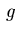in the cost functional (2.1),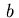in the boundary conditions (2.2), and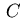in the constraints (2.3) do not depend on the state variable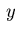. This leads to the problem of finding a control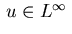that minimizes the functional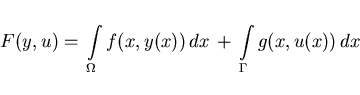(3.1)

subject to the state equation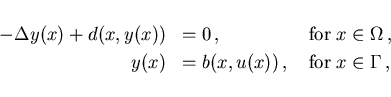(3.2)

and the inequality constraints on control and state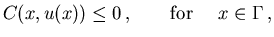(3.3)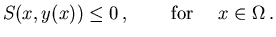(3.4)

When treating Dirichlet boundary conditions, an intrinsic difficulty arises from the fact that the solution operator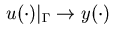for (3.2) is not sufficiently smooth as to give an appropriate form of first order necessary conditions: cf., Lions , Lions, Magenes , Chapter 2. A weak formulation of first order necessary conditions for linear elliptic equations may be found in Bergounioux, Kunisch . Instead of trying to prove first order necessary conditions under strong assumptions we content ourselves with deriving first order necessary conditions in a purely formal way. This form of the necessary conditions will be justified by its analogy in the first order necessary conditions for the discretized version of the elliptic problem; cf. Section 4.2.

Denote an optimal solution of problem (3.1)-(3.4) by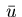and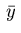. The active sets of inequality constraints (3.3) and (3.4) are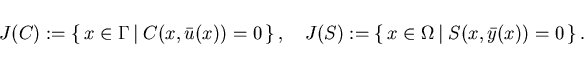We require the following regularity conditions: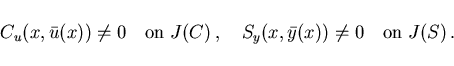(3.5)

Let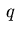and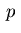be multipliers associated with the elliptic equation and the Dirichlet boundary condition in (3.2), and let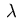resp.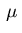be the multiplier resp. the Borel measure associated with the control and state inequality constraints (3.3) and (3.4). Then the Lagrangian for problem (3.1)-(3.4) becomes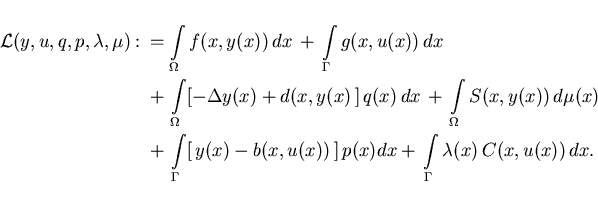The first order necessary condition with respect to the state variable gives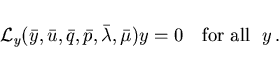Function evaluation along a stationary solution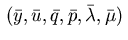will be denoted henceforth by a bar, e.g.,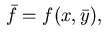etc. Using partial integration (Green's theorem),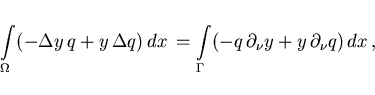we rewriteas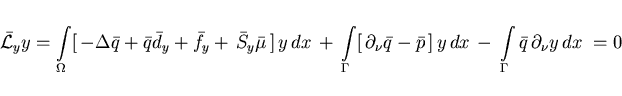which holds for all functions. From this we obtain the adjoint equations: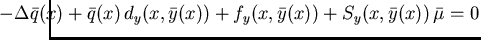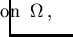(3.6)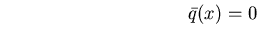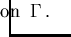(3.7)

Moreover, we find the multiplier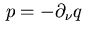on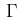. Then the optimality condition for the control variable is evaluated as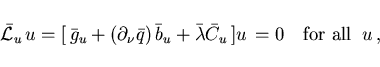which yields the minimum condition: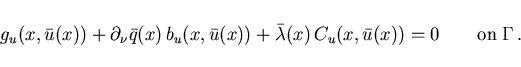(3.8)

The complementarity condition is similar to (2.10):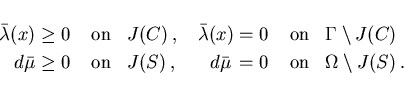(3.9)

The Borel measure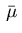has a decomposition similar to the one in (2.11).

Note that the minimum condition (3.8) agrees with the condition (2.9) if we replace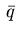formally by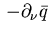. Hence, assuming functionals of tracking type (2.12), box constraints (2.13) for control and state and the property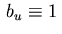, we obtain the counterparts of the control laws (2.16), (2.17).

Case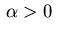: the control is determined by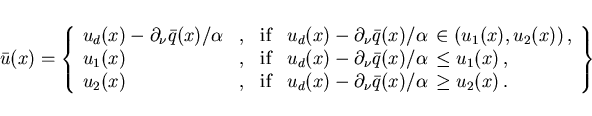(3.10)

Case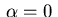: the optimal control is of bang-bang or singular type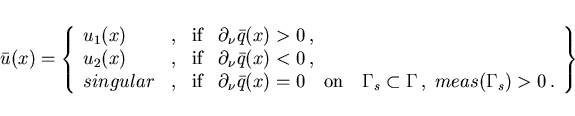(3.11)

Thus, forthe outward normal derivative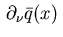on the boundary plays the role of a switching function. The isolated zeros of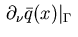are the switching points of a bang-bang control.Next: Discretization and optimization techniques Up: paper84 Previous: Elliptic control problems with
Hans D. Mittelmann
2002-11-25